# SAT II Math I : Symmetry

## Example Questions

### Example Question #1 : Symmetry

Given a point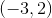, what is the newvalue if this point is flipped across the line?Explanation:

The displacement between negative three and positive one is four.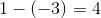This mean that after flipping the point, it must be symmetrical to its original location.   The new point must also be 4 units to the right of the line.

The new point would be located at: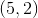The answer is:### Example Question #2 : Symmetry

Which of the following symmetries applies to the graph of the relation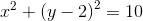?

I) Symmetry with respect to the origin

II) Symmetry with respect to the-axis

III) Symmetry with respect to the-axis

I, II and III

II only

None of these

III only

I only

III only

Explanation:

The relation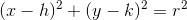is a circle with center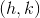and radius.

In other words, it is a circle with center at the origin, translated rightunits and upunits (the radius is irrelevant to the question).or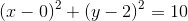is this circle translated right zero units and up 2 units. The upshot is that the circle moves along the-axis only, and therefore is symmetric with respect to the-axis, but not the-axis. Also, as a consequence, it is not symmetric with respect to the origin.